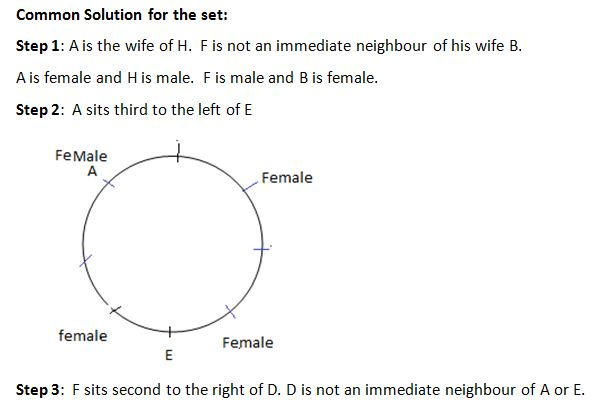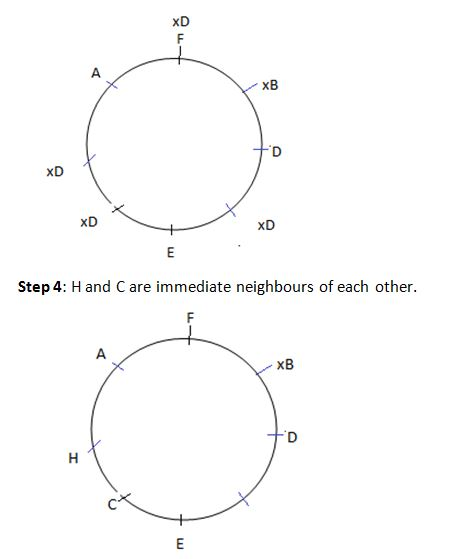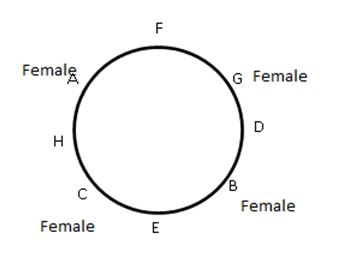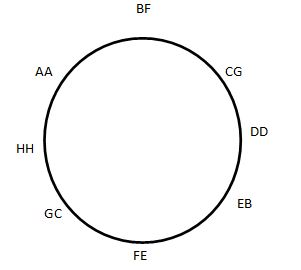### Logical reasoning sums: Logical Reasoning sums that you should solve to make your fundamentals stronger

Solving a lot of Logical reasoning sums should be given high priority while you are in the concept understanding phase. This ensures that you are thorough with your understanding of concepts and you move ahead with complete confidence. Solve a variety of Logical reasoning sums to cover all the important and commonly asked topics. Giving yourself a good practice of these sets in stipulated time will improve your speed and accuracy. Practising plenty of Logical reasoning sums can help boost your performance.

Directions for the question set:
Study the following information to answer the given questions:
A, B, C, D, E, F, G and H are sitting around a circular table facing the centre where half of them are males and half are females. No two males or two females are immediate neighbours of each other.
A is the wife of H. A sits third to the left of E. F sits second to the right of D. D is not an immediate neighbour of A or E. H and C are immediate neighbours of each other. F is not an immediate neighbour of his wife B.

Question 1: Which of the following is true about G?
(a) G is a male
(b) G sits exactly between F and H
(c) G sits third to the left of E
(d) G sits second to the right of B
(e) None is true

Question 2: Who sits third to the left of B?
(a) F
(b) H
(c) D
(d) A
(e) None of these

Question 3: How many people sit between B and F when counted in an anti-clockwise direction from B?
(a) One
(b) Two
(c) Three
(d) Four
(e) More than four

Question 4: Four of the following five are alike in a certain way and so form a group. Which is the one that does not belong to that group?
(a) H
(b) F
(c) E
(d) G
(e) D

Question 5: Which of the following groups consists of only female members of the group?
(a) A, B, H
(b) G, F, C
(c) C, H, G
(d) D, H, C
(e) None of these

Question 6: If all the persons are made to sit in alphabetical order in clockwise direction, starting from A, the positions of how many (excluding A) will remain unchanged as compared to their original seating positions?
(a) None
(b) One
(c) Two
(d) Three
(e) Four

### Answers and Explanations: Click the down arrow to expandAnswer 1: (d) G sits second to the right of B. The correct option is (d)

Answer 2: (b) H is third to the left of B. The correct option is (b)

Answer 3: (b) There are 2 people between F and B. The correct option is (b)

Answer 4: (d) G does not belong to the group. G is female. The correct option is (d)

Answer 5: (e) None of the groups given in options has only female members. The correct option is (e)

Answer 6: (c) After the rearrangement, we have the following diagram.The position of D and H remain unchanged. The correct option is (c)

Extra tips for Logical reasoning sums:
• Make sure that you use diagram constructions as soon as you understand the positions of objects/people with respect to each other.
• You should account for the multiple cases/possibilities in your diagram. It is advisable to draw parallel diagrams and immediately rule out the case that fails.
• Keep going through Logical reasoning sums from a topic till you are thorough with it.